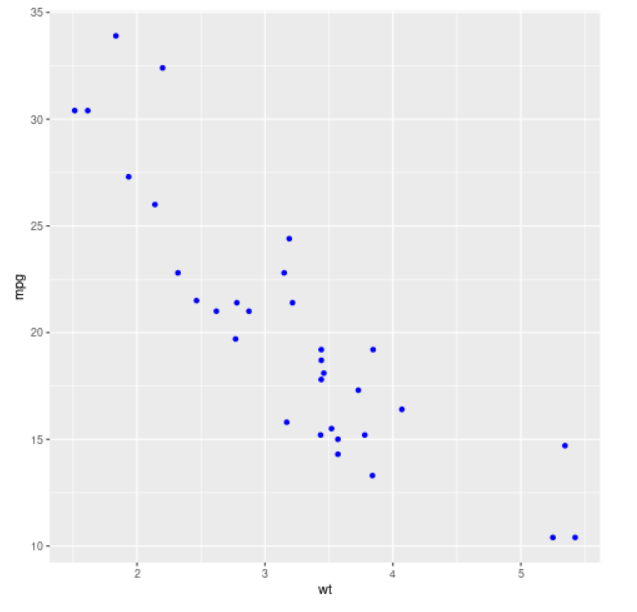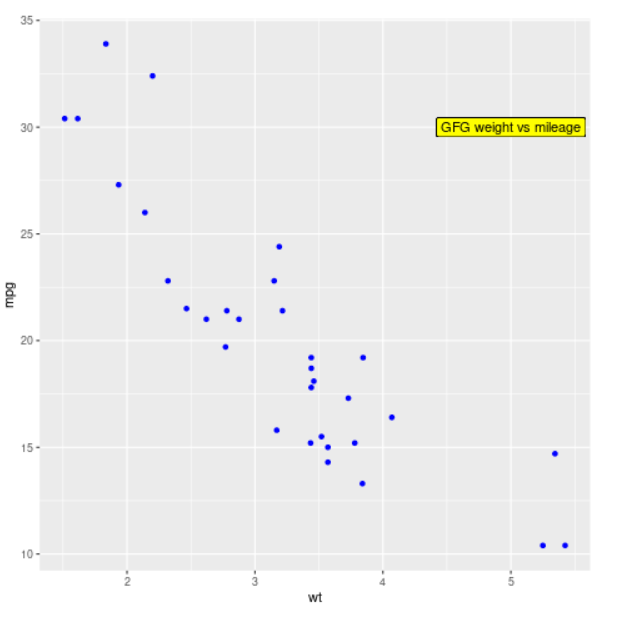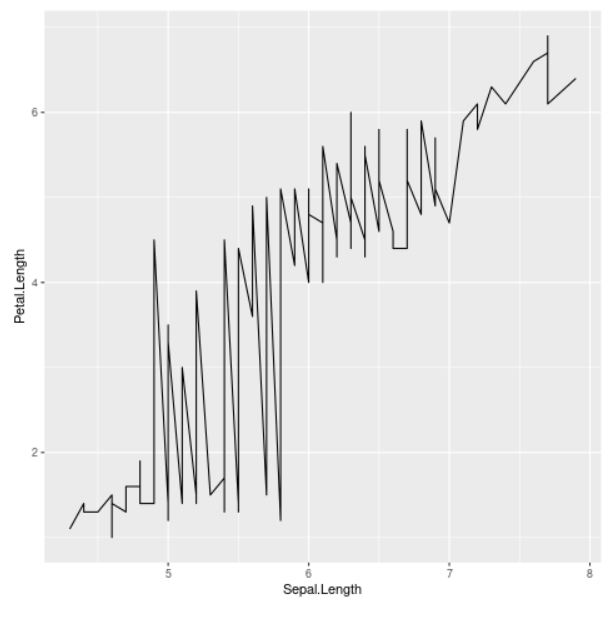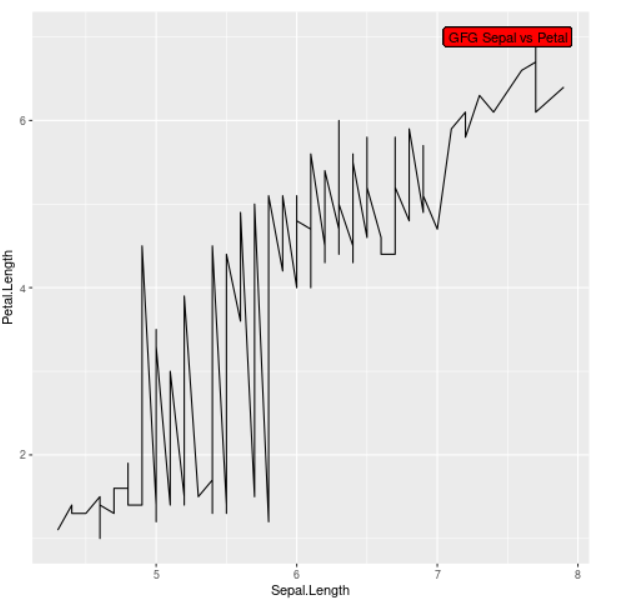# How to highlight text inside a plot created by ggplot2 using a box in R?

• Last Updated : 18 Jul, 2021

In this article, we will discuss how to highlight text inside a plot created by ggplot2 using a box in R programming language.

There are many ways to do this, but we will be focusing on one of the ways. We will be using the geom_label function present in the ggplot2 package in R. This function allows us to create a rectangle underneath the text so that it becomes easier to read. We can also highlight our text inside the rectangular box and can also add other aesthetics of that text using this function. This can be achieved by a single line of code, therefore, it is easy and recommendable.

Syntax :

geom_label( mapping = NULL, data = NULL, stat = “identity”, position = “identity”, …., parse = FALSE, nudge_x = 0, nudge_y = 0, label.r = unit( 0.15, “lines”), label.padding = unit( 0.25, “lines”), label.size = 0.25, na.rm = FALSE, inherit.aes = TRUE, show.legend = NA)

The approach is simple and straightforward, call the geom_label() function and provide the following parameters – aesthetics of the text, label of the text, and fill argument to highlight the text inside the text label.

Example 1.1: Example to show regular plot so that the difference is apparent.

## R

 `library``(ggplot2)`` ` `data``(mtcars)``head``(mtcars)`` ` `# Plotting the dataset in a scatterplot``ggplot``(mtcars, ``aes``(wt, mpg)) + ``geom_point``(color=``"blue"``)`

Output :Now, we will add text and highlight it in our scatterplot using the geom_label function taking the aesthetics of the text such as x and y variables, label of the text, and color of the highlighted text inside the box as its arguments.

Example 1.2: Example to plot highlight text inside the plot

## R

 `library``(ggplot2)`` ` `data``(mtcars)``head``(mtcars)`` ` `# Plotting the data, adding the text and highlighting it``ggplot``(mtcars, ``aes``(wt, mpg)) + ``geom_point``(color=``"blue"``) + ``        ``geom_label``(``aes``(x=5, y=30, label = ``"GFG weight vs mileage"``),``                   ``fill= ``"yellow"``)`

Output :Let us see one more implementation.

Example 2.1: Regular plot, so that the difference is apparent

## R

 `library``(ggplot2)`` ` `data``(iris)``head``(iris)`` ` `# Line plot``ggplot``(iris, ``aes``(Sepal.Length, Petal.Length)) + ``geom_line``()`

Output :Now, we will add text and highlight it in our line plot using the geom_label function providing the aesthetics of the text as arguments.

Example 2.2: Example to plot highlight text inside the plot

## R

 `library``(ggplot2)`` ` `data``(iris)``head``(iris)`` ` `# Adding the text and highlighting it``ggplot``(iris, ``aes``(Sepal.Length, Petal.Length)) + ``geom_line``() + ``            ``geom_label``(``aes``(x=7.5, y=7, label = ``"GFG Sepal vs Petal"``),``                       ``fill= ``"red"``)`

Output :My Personal Notes arrow_drop_up